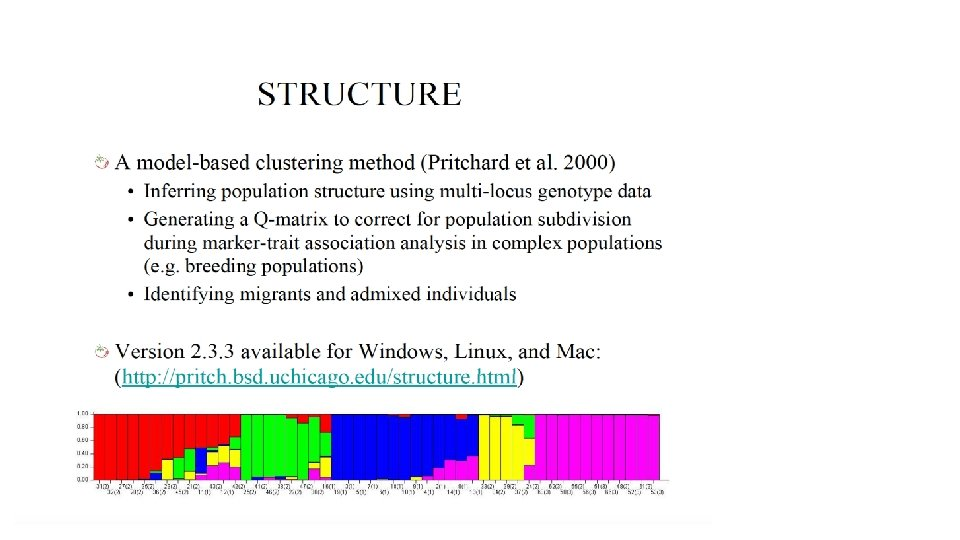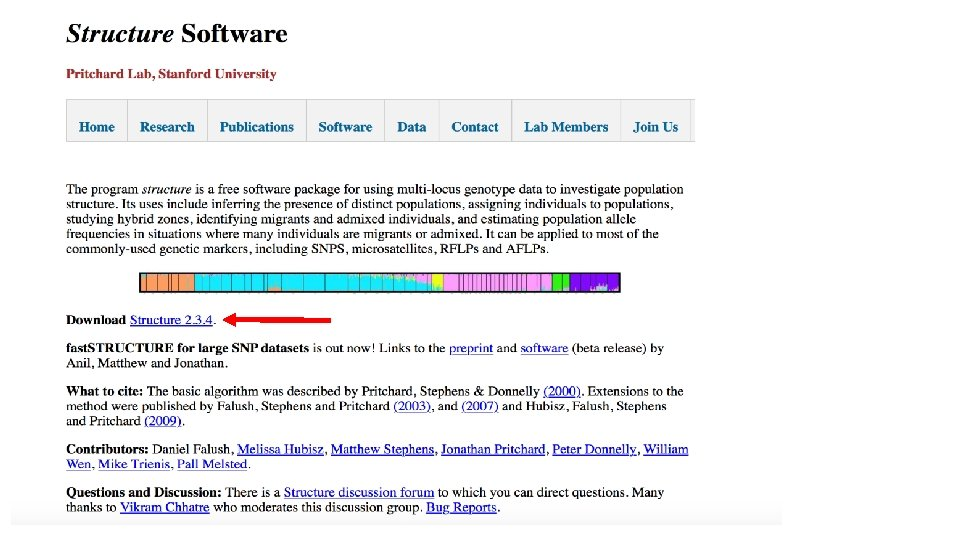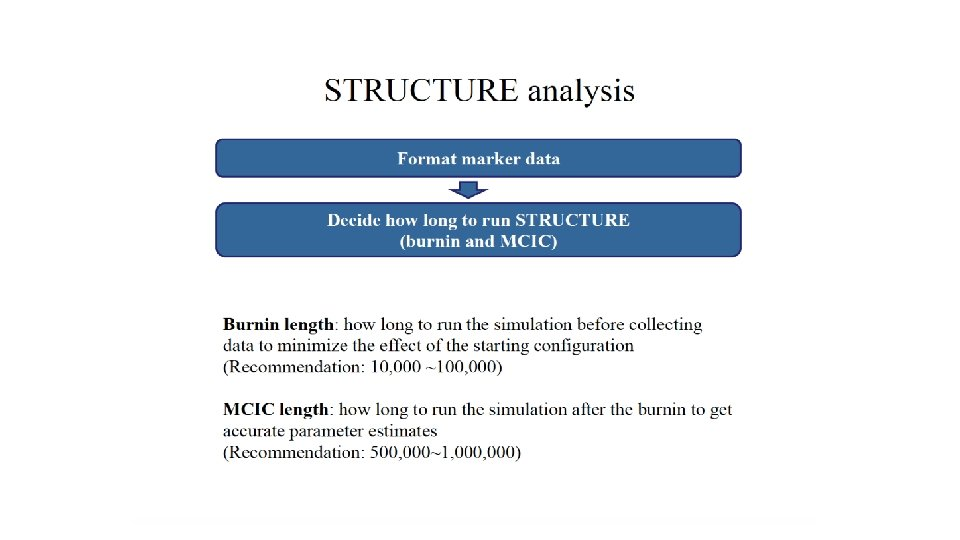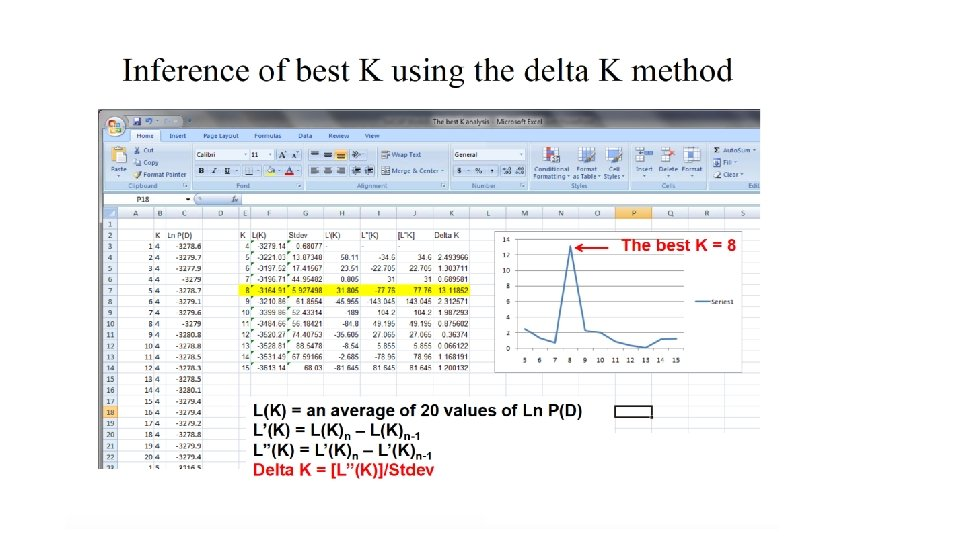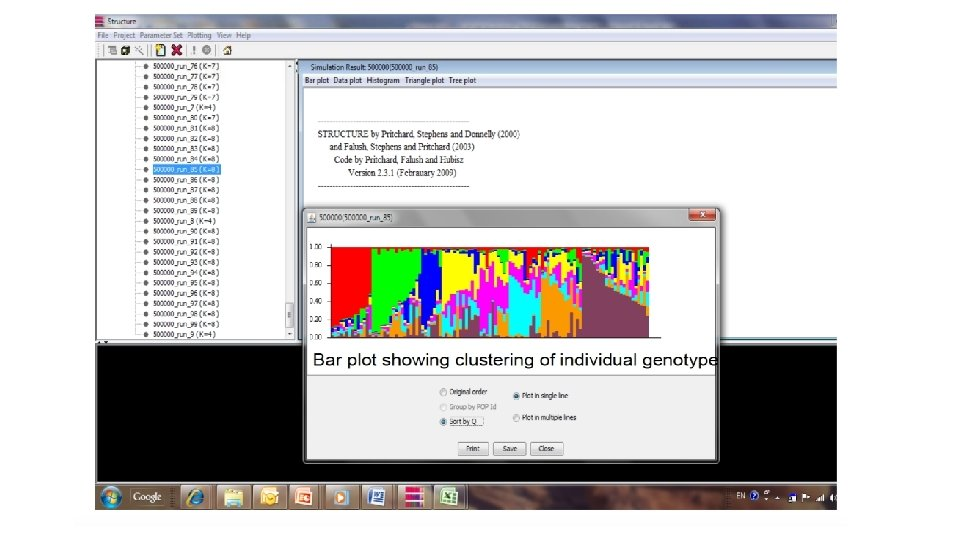# Measure of diversity 1 Diversity Indices A diversity

• Slides: 17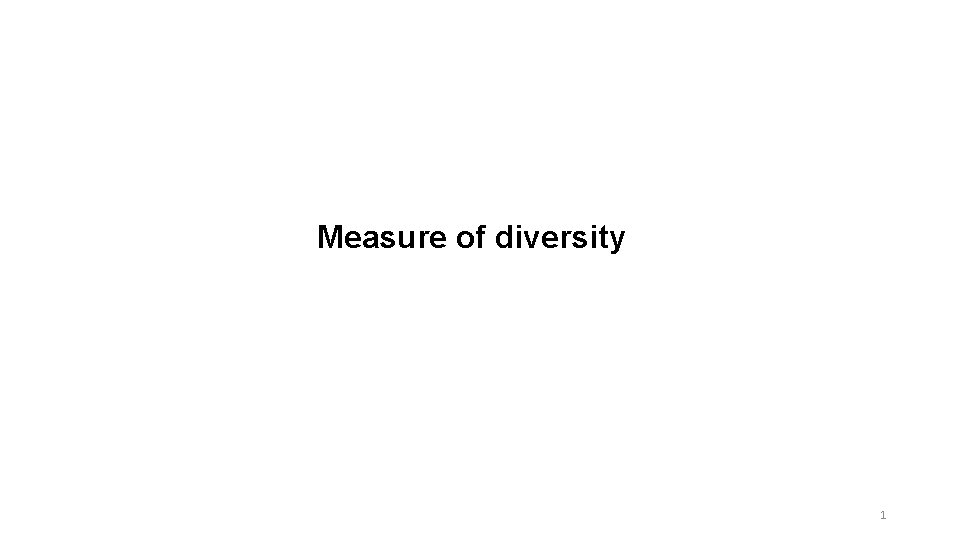Measure of diversity 1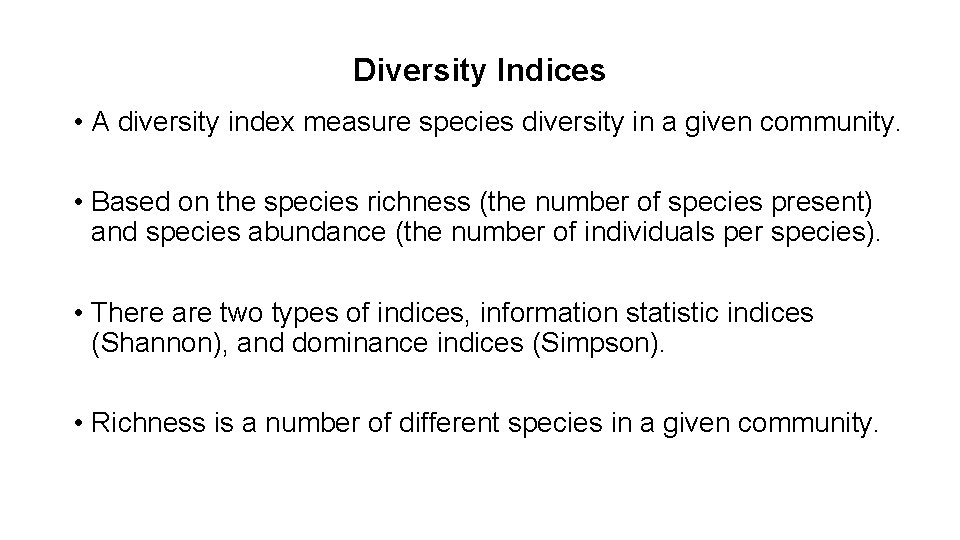Diversity Indices • A diversity index measure species diversity in a given community. • Based on the species richness (the number of species present) and species abundance (the number of individuals per species). • There are two types of indices, information statistic indices (Shannon), and dominance indices (Simpson). • Richness is a number of different species in a given community.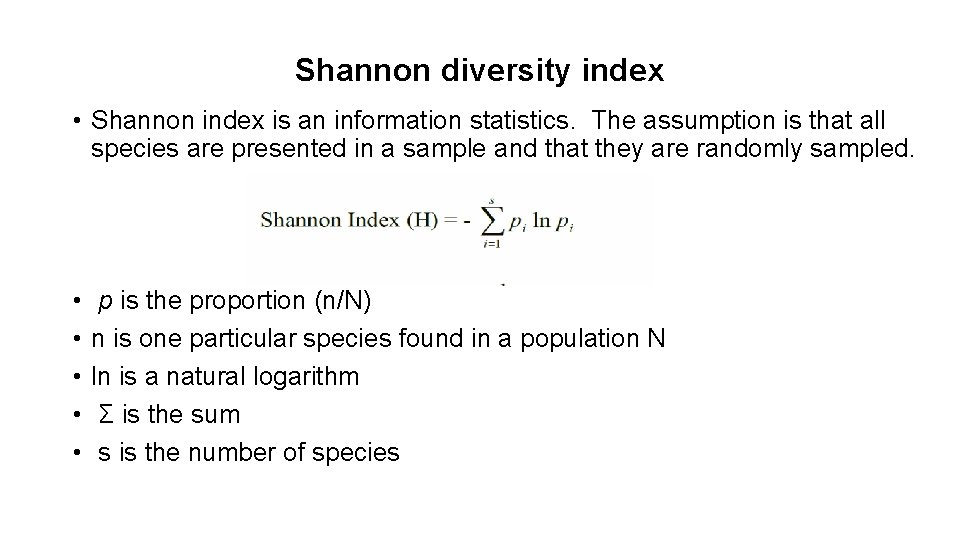Shannon diversity index • Shannon index is an information statistics. The assumption is that all species are presented in a sample and that they are randomly sampled. • • • p is the proportion (n/N) n is one particular species found in a population N ln is a natural logarithm Σ is the sum s is the number of species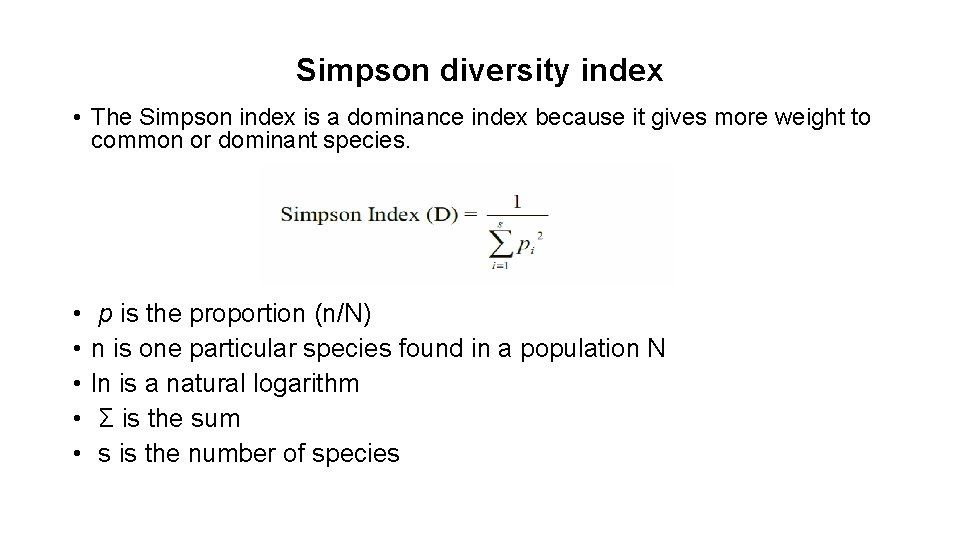Simpson diversity index • The Simpson index is a dominance index because it gives more weight to common or dominant species. • • • p is the proportion (n/N) n is one particular species found in a population N ln is a natural logarithm Σ is the sum s is the number of species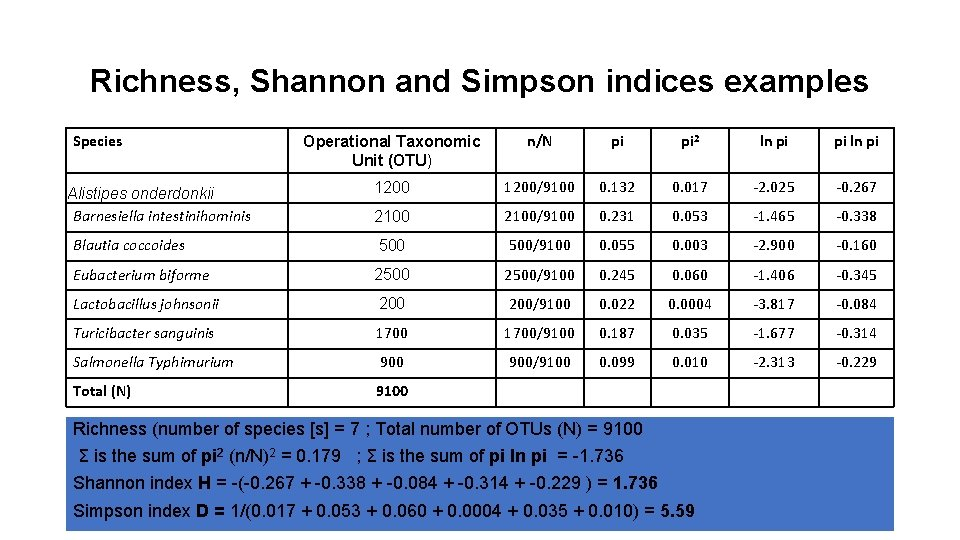Richness, Shannon and Simpson indices examples Species Operational Taxonomic Unit (OTU) n/N pi pi 2 ln pi pi ln pi 1200/9100 0. 132 0. 017 -2. 025 -0. 267 2100/9100 0. 231 0. 053 -1. 465 -0. 338 Blautia coccoides 500/9100 0. 055 0. 003 -2. 900 -0. 160 Eubacterium biforme 2500/9100 0. 245 0. 060 -1. 406 -0. 345 Lactobacillus johnsonii 200/9100 0. 022 0. 0004 -3. 817 -0. 084 Turicibacter sanguinis 1700/9100 0. 187 0. 035 -1. 677 -0. 314 Salmonella Typhimurium 900/9100 0. 099 0. 010 -2. 313 -0. 229 Total (N) 9100 Alistipes onderdonkii Barnesiella intestinihominis Richness (number of species [s] = 7 ; Total number of OTUs (N) = 9100 Σ is the sum of pi 2 (n/N)2 = 0. 179 ; Σ is the sum of pi ln pi = -1. 736 Shannon index H = -(-0. 267 + -0. 338 + -0. 084 + -0. 314 + -0. 229 ) = 1. 736 Simpson index D = 1/(0. 017 + 0. 053 + 0. 060 + 0. 0004 + 0. 035 + 0. 010) = 5. 59 5STRUCTURE